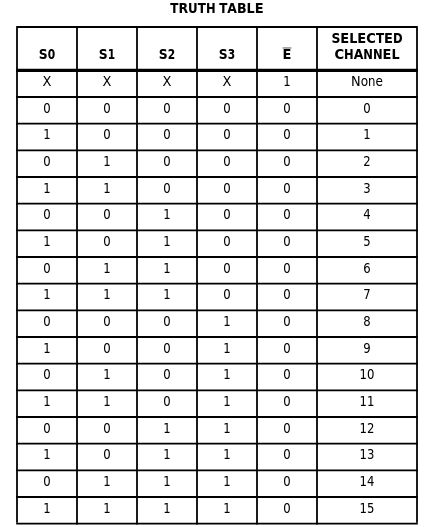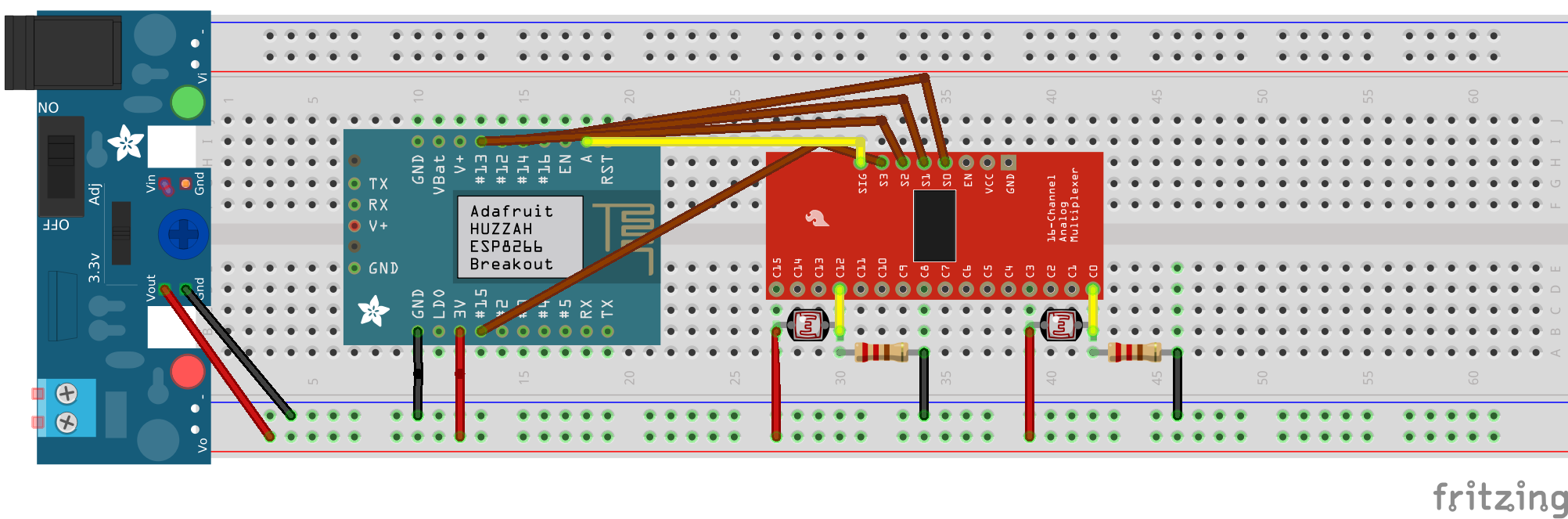# Why use a multiplexer?¶

One disadvantage of the tiny WiFi-capable `ESP8266` micro-controller is its single analog input pin. This makes it impossible to measure, for instance, multiple photo-resistors at once. To solve this issue we experimented with using a 1-to-16 channel multiplexer known as `CD74HC4067`. This particular multiplexer let’s you read from 16 different possible analog devices by switching between them. Like all microchips, the idea behind the switch is to use a logic scheme to change between the channels. To control the `CD74HC4067` you need to sacrifice 5 of the `GPIO` pins. Here’s the logic table obtained from the Texas instruments website:# Wiring¶

Here’s how to wire the `ESP8266` to one of these multiplexers:In this example we connected 2 photoresistors to 2 different analog channels (0 and 12).

# Micropython for CD74HC4067¶

We wrote a micropython class for `ESP8266` (Huzzah Feather in particular) to be able to easily control and read from the analog channels. Let’s go through it step by step:

We first use micropython’s machine module to control the output from 5 `GPIO` pins of choice and the single analog input:

``````import machine

class Mux:
def __init__(self, S0, S1, S2, S3, E, signal=0):
self.S0 = machine.Pin(S0, machine.Pin.OUT)
self.S1 = machine.Pin(S1, machine.Pin.OUT)
self.S2 = machine.Pin(S2, machine.Pin.OUT)
self.S3 = machine.Pin(S3, machine.Pin.OUT)
self.E = machine.Pin(E, machine.Pin.OUT)
self._reset()
self.current_bits = "0000"
self.state = False
``````

Now we are capable of controlling the output pins to change the channel according to the logic table. It’s best practice to create a method to reset all the `S` pins to `0` at first and the `E` pin to `1` inside the init. We can add a `_reset()` function like this to do it:

``````    def _reset(self):
self.S0.off()
self.S1.off()
self.S2.off()
self.S3.off()
self.E.off()
``````

Then we can write a function to simply flip the `E` state on or off to enable/disable the multiplexer when needed:

``````    def switch_state(self):
if self.state:
self.E(0)
self.state = False
else:
self.E(1)
self.state = True
``````

Now we need a function to map the channel id to bits and vice versa:

``````    def _bits_to_channel(self, bits):
return int("0000" + "".join([str(x) for x in "".join(reversed(bits))]), 2)

def _channel_to_bits(self, channel_id):
return ''.join(reversed("{:0>{w}}".format(bin(channel_id)[2:], w=4)))
``````

The trick to the `bits_to_channel` function is to concatenate the 4 logic bits with a filler of `0000` string and convert it to its corresponding `8bit` integer value. Ah, another detail is to also flip the 4 bits (for unknown reasons). Of course, this mapping can also written out and hard coded if you don’t mind the looks. For the `channel to bits` function, we used python’s built in bin function to first obtain the 8bit string for the channel id and then reverse it before returning. A side note: reversing a list by `alist[::-1]` does not work in micropython.

Now we need to have a function for switching the pins according to the given channel specific 4bit value:

``````    def _switch_pins_with_bits(self, bits):
s0, s1, s2, s3 = [int(x) for x in tuple(bits)]
self.S0(s0)
self.S1(s1)
self.S2(s2)
self.S3(s3)
``````

Lastly, the most important function takes a channel ID and switches the analog input to that:

``````    def switch_channel(self, channel_id):
bits = self._channel_to_bits(channel_id)
self._switch_pins_with_bits(bits)
self.current_bits = bits
``````

# Example usage¶

After we put this class into the `boot.py` file, we can transfer it to the esp8266 and reboot the board.

Then we can turn on the `REPL` to switch channels and read the signal:

``````mux = Mux(14, 12, 13, 15, 16)       # this is according to the GPIO wiring used.
mux.switch_state()                 # turns on the reading
print(mux.signal.read())            # reads the signal from channel 0 (default channel → “0000”)
mux.switch_channel(12)             # switches to channel 12